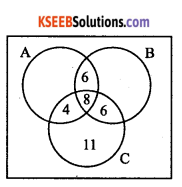# 1st PUC Maths Question Bank Chapter 1 Sets

Students can Download Maths Chapter 1 Sets Questions and Answers, Notes Pdf, 1st PUC Maths Question Bank with Answers helps you to revise the complete Karnataka State Board Syllabus and score more marks in your examinations.

## Karnataka 1st PUC Maths Question Bank Chapter 1 Sets

Question 1.
Define a set.
A collection of well-defined objects is called a set. The objects in a set are called its members or elements. We denote sets by capital letters A, B, C, X. Y, Z, etc. If ‘a’ is an element of a set A, we write, a ∈ A, which means that a belongs to A or that a is an element of If ‘a ’ does not belong to A, we write, a ∉ A

Question 2.
How to describe a set?
There are two methods of describing a set.
1. Roster or tabular form: In the roster form, we list all the members of the set within brackets { } and separate them by commas.

2. Set-Builder form: In the set-builder form, we list the property or properties satisfied by all the elements of the set.

• N: The set of all-natural numbers.
• Z: The set of all integers.
• Q: The set of all rational numbers.
• M: The set of real numbers.
• Z+: The set of positive integers.
• Q+: Set of positive rational numbers.
• R+: The set of positive real numbers.Question 3.
(i) The collection of all the months of a year beginning with the letter.
(ii) The collection of ten most talented writers of India.
(iii) A team of eleven best-cricket batsmen of the world.
(iv) The collection of all boys in your class.
(v) The collection of all natural numbers less than 100.
(vi) A collection of novels written by the writer Munshi Prem Chand.
(vii) The collection of all even integers.
(viii) The collection of questions in this chapter.
(ix) A collection of most dangerous animals of the world.
(i) The given collection is {January, June, July}, which consists of definite objects. So, it is a set.
(ii) The term most talented is vague. So, this collection is not a set.
(iii) The term best is vague. So, this collection is not a set.
(iv) This collection is well defined and hence is a set.
(v) This collection is well defined and hence it is a set.
(vi) The collection of novels written by Munshi Prem Chand is well defined, so it is a set.
(vii) {0,±2,±4,±6,—} is the collection of well-defined objects, so it is a set.
(viii) The collection of questions in this chapter is well defined, so it is a set.
(ix) The term most dangerous is vague. So, this collection is not a set.

Question 4.
Let A = {1, 2, 3,4,5, 6}. Insert the appropriate symbol 6 or g in the blank spaces:
(i) 5 ……………. A
(ii) 8…………….. A
(iii) O……………. A
(iv) A ……………. A
(v) 2……………. A
(vi) 10 ……………. A
(i) 5 ∈ A
(ii) 8 ∉ A
(iii) 0 ∉ A
(iv) 4 ∈ A
(v) 2 ∈ A
(vi) 10 ∉A

Question 5.
Write the following sets in roster form:
(i) A = {x : x is an integer and – 3 < x < 7}
(ii) B = {x : x is a natural number less than 6}
(iii) C = {x : x is a two-digit natural number such that the sum of its digits is 8}
(iv) D =  {x : x is a prime number which is divisor of 60}
(v) E = The set of all letters in the word TRIGONOMETRY.
(vi) F = The set of all letters in the word BETTER.
(vii) G = The solution set of the equation x2 + x-2 = 0.
(viii) H = {x : x is a positive integer and x2 < 40}
(i) A = {-2,-1,0,1,2,3,4,5,6}
(ii) B = {1,2,3,4,5}
(iii) C = {17,26,35,44,53,62,71,80}
(iv) D = {2,3,5}
(v) E = {T,R,I,G,0,N,M,E,Y}
(vi) F = {B,E,T,R}
Given equation is x2 + x – 2 = 0
⇒ (x – 1)(x + 2) = 0
i.e., = 1,-2
∴ G = { 1,-2}
(viii) H ={1,2,3,4,5,6}

Question 6.
Write the following sets in the set-builder form
(i) {3,6,9,12}
(ii) {2,4,8,16,32}
(iii) {5,25,125,625}
(iv) {2,4,6,… }
(v) {1,4,9,…, 100}
(vi) {1,4,9,16,…}
(vii) $$\frac { 1 }{ 2 } ,\frac { 2 }{ 3 } ,\frac { 3 }{ 4 } ,\frac { 4 }{ 5 } ,\frac { 5 }{ 6 } \frac { 6 }{ 7 }$$
The given sets in set – builder form are
(i) {x : x = 3n, n∈N,n<5}
(ii) {x : x = 2n,n∈N,n<6}
(iii) {x : x = 5n,n∈ N, n<5}
(iv) {x : x is an even natural number}
(v) {x : x = n2,n∈ N,n≤10}
(vi) {x : x = n2,n∈ N}
(vii)
$$\left\{x: x=\frac{n}{n+1}, n \in N, n<7\right\}$$

Question 7.
List all the elements of the following sets:
(i) A = {x : x is an odd natural number}
(ii) B = { x : x is an integer, $$-\frac { 1 }{ 2 } <x<\frac { 9 }{ 2 }$$
(iii) C = {x : x is an integer, x2 ≤ 4}
(iv) D = {x : x is a letter in the word “LOYAL”}
(v) E = { x : x is a month of a year not having 31 days}
(vi) F =  {x : x is a consonant in the English alphabet which precedes k}
(i) A = {1,3,5,.. }
(ii) B = {0,1,2,3,4}
(iii) C = {-2,-1,0,1,2}
(iv) D = {L,0,Y,A}
(v) E = {February, April, June, September,November}
(vi) F = {b,c,d,f,g,h,j}

Question 8.
Match each of the set on the left in the roster form with the same set on the right described in set-builder form:

 (i) (1,2,3,6} (a) (x : x is a prime number and ‘ a divisor of 6} (ii) {2,3} (b) {x : x is an odd natural number less than 10} (iii) {M, A, T, H, E, I,C,S} (c) {x : x is a natural number and divisor of 6} (iv) 11,3,5,7,9} (d) {x : x is a letter of the  word MATHEMATICS}

(i) →(c), (ii) → (a), (iii) → (d), (iv) → (b).

Question 9.
Match each of the set of on the left described in the roster form with the same set on the right described in the set – builder form.

(i) → (d), (ii) → (c), (iii) → (a), (iv) → (b).

Question 10.
Define an empty set.
A set which does not contain any element is called the empty set or the null set or the void set and it is denoted by { } or φ.

Question 11.
Define a finite set.
A set which is empty or consists of a definite number of elements is called finite set.

Question 12.
Define an infinite set.
A set having infinite elements is called an infinite set.Question 13.
Which of the following are examples of the null set.
(i) Set of odd natural numbers divisible by 2.
(ii) Set of even prime numbers.
(iii) {x : x is a natural numbers, x < 5 and x >7}
(iv) { y: y is a point common to any two parallel lines}.
(i) There is no odd natural number divisible by 2. Therefore, given set is an empty set / nullset.
(ii) 2 is the even prime number.
∴ Given set = {2} ≠φ
(iii) There is no natural number x, which is simultaneously less than 5 and greater than 7. So, given set is null set.
(iv) We know that two parallel lines have no common point and hence the given set is null set.

Question 14.
Which of the following sets are finite or infinite?
(i) The set of months of a year,
(ii) {1, 2, 3, ….}
(iii) {1, 2, 3, …….99, 100}
(iv) The set of positive integers greater than 100
(v) The set of prime numbers less than 99.
(i) Finite set
(ii) Infinite set
(iii) Finite set
(iv) Infinite set
(v) Finite set.

Question 15.
State which of the following sets are finite or infinite.
(i) {x : x ∈ N and (x – 1)(x – 2) = 0}
(ii) {x : x ∈ N and x2 = 4}
(iii) {x : x ∈ N and 2x-1 = 0}
(iv) {x : x ∈ N and x is prime}
(v) {x : x ∈ N and x is odd}
(vi) The set of lines which are parallel to the x-axis.
(vii) The set of letters in the English alphabet.
(viii) The set of numbers which are multiple of 5.
(ix) The set of animals on the earth
(x) The set of circles passing through the origin (0,0).
(i) Given set = {1,2}. Hence, it is finite.
(ii) Given set = {2}. Hence, it is finite.
(iii) Given set = φ. Hence, it is finite.
(iv) We know that there are infinitely many primes. Hence, given set is infinite.
(v) There are infinitely many odd numbers. Hence, given set is infinite set.
(vi) There are infinitely many parallel lines to the X-axis. Hence, given set is infinite set.
(vii) Finite sets
(viii) Infinite set
(ix) Finite set
(x) Infinite set.

Question 16.
Define equal sets.
Two sets A and B are said to be equal if they have exactly the same elements and we write A = B.Question 17.
In the following, state whether A = B or not.
(i) A = {a,b,c,d}, B={d,c,b,a}.
(ii) A = {4,8,12,16}, B = {8,4,16,18}.
(iii) A = {2,4,6,8,10}, B ={x : x is positive even integer and x ≤ 10}.
(iv) A = {x : x is a multiple of 10}, B={10,15,20,25,30,-}.
(v) A = {x: x is a prime numbers ≤ 6},
B = {x: x is a prime factors of 30}.
(i) A = B because A and B have same elements though in different order which is immaterial.
(ii) A≠B ∵ 12 ∈ A but 12 ∉ B
(iii) A = B ∵ A and B have same elements
(iv) A≠B ∵ 15 ∈ B
(v) A = {2,3,5}, B = {2,3,5} ∴ A = B.

Question 18.
Are the following pairs of sets equal? Give reasons.
(i) A = {2,3}, B = {x : x is solution of x2+5x + 6 = 0}.
(ii) A = {x : x is a letter in the word FOLLOW}.
B={y: y is a letter in the word WOLF}.
(i) x2 + 5x + 6 = 0
⇒ (x + 3)(x + 2) = 0
∴ x = -3,-2
∴B = {-3,-2}- But A = {2,3}
∴ A≠B(ii) Given sets are A = {F, O, L, W}
B = {W,0,L,F}
∴ A = B
∵ A and B have same elements.

Question 19.
From the sets given below, select equal sets.
A = {2,4,8,12}
B = {1,2,3,4}
C ={4,8,12,14}
D ={3,1,4,2}
E = {-1,1}
F={0,a}
G = {1,-1}
H ={0,1}.
Here, B = D and E = G.

Question 20.
Define subset of a set.
A set A is said to be subset of set B if every element of A is also an element of B, and we write, A ⊆ B

• If A ⊆ B then B is called a superset of A, and we write, B ⊇ A
• If A ⊆ B and A ≠ B then A is called a proper subset of B.
• If there exists even a single element in A which is not in B, then A is not a subset of B and we write, A⊄B
• Every set A is a subset of itself
• Empty set φ is a subset of every set.

Question 21.
Consider the sets φ, A={1,3},B{1,5,3},C ={1,3,5,7,9}. Inset the symbol ⊂ or ⊄ between each of the following pair of sets:
(i) φ……… B
(ii) A … B
(iii) A… C
(iv) B… C.
(i) φ ⊂ B, as empty set is a subset of every set
(ii) A ⊄ B, ∵ 3∈  A ⇒ but 3∉ B
(iii) A⊂C, as 1,3∈ A ⇒1,3 ∈ C
(iv) B⊂C, as 1,5,9∈ A ⇒ 1,5,9 ∈ C.

Question 22.
Make correct statements by filling in the symbols ⊂ or ⊄ in the blank spaces:
(i) {2,3,4} … {1,2,3,4,5}.
(ii) {a, b, c} … {b,c,d}.
(iii) {x : x is a circle in the plane } … {x : x is a circle in the same plane with radius 1 unit}.
(iv) {x : x is a student of I PUC of your college} … {x : x is student of your college}.
(v) {x : x is a triangle in a plane } … {x: x is a rectangle in the plane}.
(vi) {x : x is an equilateral triangle in a plane} … { x : x is a triangle in the same plane}.
(vii) {x : x is an even natural number} … {x : x is an integer}.
(i) {2,3,4} ⊂ {1,2,3,4,5} ∵ 2,3,4 ∈{1,2,3,4,5}

(ii) {a,b,c}⊄ {b,c,d} ∵ a ⊄ {b,c,d}

(iii) {x: x is a circle in the plane} ⊄ {x: x is a circle in the same plane with radius 1 unit}. Because there exists a circle having a radius other than 1 unit.

(iv) {x: x is a student of 1 PUC of your college} ⊄ {x: x is a student of your college}
∵ Every student of I PUC is a student of the college.

(v) {x: x is a triangle in a plane} ⊄ {x: x is a rectangle in the plane.
∵ Triangle is not a rectangle.

(vi) {x: x is an equilateral triangle in a plane} ⊂ {x: x is a triangle in the same plane}.
∵ Every equilateral triangle in a plane is a triangle in the same plane.

(vii) {x : x is an even natural number} ⊂ {x : x is an integer}
∵ Every even natural number is an integer.Question 23.
Examine whether the following statements are true or false:
(i) {a, b} ⊄ {b,c,a}
(ii) {a,e} ⊂ {x:x is a vowel in the English alphabet}
(iii) {1,2,3} ⊂ {1,3,5}
(iv) {a} ⊂ {a,b,c}
(v) {a}∈{a,b,c}
(vi) {x: x is an even natural number less than 6} ⊂ {x : x is a natural number which divides 36}.
(i) False ∵ a,b∈ {b,c,a}
(ii) True ∵ a,e∈ {a,e,i,o,u}
(iii) False ∵ 2∉{1,3,5}
(iv) True ∵ a∈ {a,b,c}
(v) False ∵ {a} is a set but not in [a, b,c}
(vi) True ∵ {2,4} ⊂ {1,2,3,4,6,9,12,18,36}

Question 24.
Let A = {1, 2, {3,4}, 5}. Which of the following statements are incorrect and why?
(i) {3,4} ⊂ A
(ii) {3,4} ∈ A
(iii) {{3,4}} ⊂ A
(iv) 1 ∈ A
(v) 1⊂ A
(vi) {1,2,5} ⊂ A
(vii) {1,2,5} ∈ A
(viii) {1,2,3} ⊂ A
(ix) φ ∈ A
(xi) {φ} ⊂ A
(x) φ ⊂A
(i) Incorrect, ∵ 3∈ {3,4} but 3∉ A.
(ii) Correct, ∵ {3,4} is a member of A.
(iii) Correct, ∵ {3,4}∈ A.
(iv) Correct, ∵  1 is a member of A.
(v) Incorrect, ∵ 1 is  A but not 1⊂ A.
(vi) Correct, ∵ 1, 2, 5, ∈ A.
(vii) Incorrect, ∵ {1,2,5}∉A.
(viii) Incorrect, ∵ 3∈ {1,2,3} but 3∉A
(ix) Incorrect, ∵ φ ∉ A
(x) Correct, ∵ φ is a subset of every set.
(xi) Incorrect, ∵ φ ∈  {φ} and φ ∈ A.

Question 25.
Define a power set of set.
The collection of all subsets of a set A is called the power set of A and is denoted by
P (A). If n(A) = m, then n[P(A)] = 2m.Question 26.
Define the universal set.
If there are some sets under consideration, then there happen to be a set which is a super set of each one of the given sets. Such a set is known as the universal set for those sets. The universal set is denoted by U.Question 27.
Write down all the subsets of the following sets:
(i) {a}
(ii) {a, b}
(iii) {1,2,3}
(iv) (-1,0,1}
(i) Subsets of {a} are {a}, φ
(ii) Subsets of {a, b} are {a, b},φ,{a},{b}
(iii) Subsets of {1, 2, 3} are {1, 2, 3}, are {1}, {2}, {3}, {1,2}, {2, 3}, {3,1}
(iv) Subsets of {-1, 0, 1} are {-1, 0, 1}, φ, {-1}, {0}, {1}, {-1,0}, {0,1}, {-1,1}

Question 28.
How many elements has P(A), if A =φ?
P(A) has only one element, namely φ.
∴ PW = {φ}

Question 29.
What universal set (s) would you propose for each of the following:
(i) The set of right triangles.
(ii) The set of isosceles triangles.
(i) Given set is the set of right triangles, so our proposed universal set is the set of all triangles.
(ii) Given set is the set of isosceles triangles, so our proposed universal set is the set of all triangles.Question 30.
Given the sets A = {1, 3, 5}, B = {2, 4, 6} and C = {0, 2, 4, 6, 8}, which of the following may be considered as universal set (s) for the three sets A, B, and C.
(i) {0, 1, 2, 3, 4, 5, 6}
(ii) ϕ {0,1, 2,3,4,5, 6, 7, 8, 9,10}
(iii) {0,1,2,3,4,5,6 7, 8, 9,10}
(iv) {1, 2,3, 4, 5,6,7,8}
Here universal setQuestion 31.
Write the following as intervals:
(i) {x: x ∈ R,-4< x ≤6},
(ii) {x:x ∈ R,-12<x <-10}
(iii) {x:x∈ R,0≤x<7}
(iv) {x:x∈ R,3≤x≤4}.
(i) (-4,6]
(ii) (-12,-10)
(iii) (0,7)
(iv) [3,4]

Question 32.
Write the following intervals in set – builder form:                                 ,
(i) (-3,0)
(ii) [6,12]
(iii) (6,12]
(iv) [-23,5).
(i) (-3,0)={x: x ∈ R, -3 < x < 0}
(ii) [6,12]={x:x ∈ R,6<x≤12]
(iii) (6,12]={x:x ∈ R,6<x≤12}
(iv) [-23,5)=[x: x ∈ R,-23≤x< 5}.

Question 33.
Decide, among the following sets, which sets are subsets of one and another:
A = {x: x ∈ R and satisfy x2 – 8x +12 = 0},
B ={2,4,6}
C={2,4,6,8,-}
D = {6}.
x2-8x + 12 = 0
⇒(x-6)(x-2) = 0
⇒ x = 2,6
∴ A = {2,6}
∴ A ⊂ B and A ⊂ C; B ⊂ C, D ⊂ B and D ⊂ C.

Question 34.
In each of the following, determine whether the statement is true or false. If it is true, prove it. If it is false, give an example.
(i) If x ∈ A and A∈B, then x∈B
(ii) If A ⊂ B and BC, then A ∈ C
(iii) If A⊂ B and B⊂ C, then A ⊂ C
(iv) If A ⊄ B and B ⊄C,then A ⊄C
(v) If x ∈A and A⊄B, then x ∈ B
(vi) If A ⊂ B and x∈B, then x ∉A
(i) False: For example, let A = {1, 2} and
B = (2, 3, {1, 2}}. As 1∈A and A∈ B but 1∉ B

(ii) False: For example, let A = {1,2},B = {1,2,3} and C = {3,4,{1,2,3}}
Now clearly, A ⊂ B and B⊂C but A∉C,

(iii) True: Given A ⊂ B and
Now we have to prove A ⊂ C
∴ x∈C ∵ B∈C.
∀ X∈A ⇒X∈C∴ A∈C.(iv) False: For example, let
A=(1,2), B={2,3),C=(1,2,4)
Clearly A⊄B and B⊄C but A⊂C(v) False: For example, let
A=(l,2), B={2,3,4,5}
Now 1 ∈A and A ∉ B but 1 ∉ B(vi) True: Suppose x∈A then X∈ B A⊂B.

Question 35.
If A⊂B, then show that C-B⊂C-A
Let x∈C-B ⇒x∈C but x ∉ B
⇒x∈C but x∉A ∵ A⊂B
∴ x∈C- A
Thus, C-B⊂C-AQuestion 36.
Assume that P(A) P(B). Show that A – B
Let x∈A∴(X)∈P(A)
={x}∈P(B) ∵ P(A)=P(B)
∴ x∈ B.
∴ A⊂B
Let y∈ B ∴(y)∈ P(B)
(y)∈P(A) ∵ P(A)=P(B)
y∈A
B⊂A
From(1) and (2),we get A=B

Question 37.
Define union of sets.
The union of two sets A and B denoted by A∪B, is the set of all those elements which arc either in A or in B or in both A and B.
Thus, AB=(x: x∈A or x∈B)
∴ x∈A∪B x∈A or x∈B
x∉A∪B=x∉A and z∉B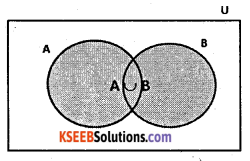• A∪B=B∩A (Commutative Law)
• (A∪B)∪C=A∪(B∪C) (Associative law)
• A∪φ=A
• A∪A=A
• U∪A=U

Question 38.
Define intersection of sets.
The intersection of sets A and B is the set of all elements which are common to both A and B and is denoted by A∩B. Thus, A∩B = {x;x∈ A and x∈ B}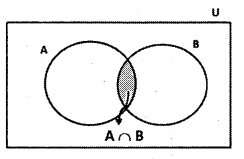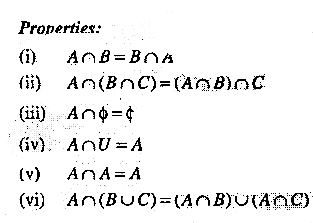Question 39.
Define disjoint sets.
If A and B are two sets such that A∩B =φ, then A and B are called disjoint sets.

Question 40.
Define difference of sets.
The difference of the sets A and B i.e., A – B. is the set of all the elements of A but not in B.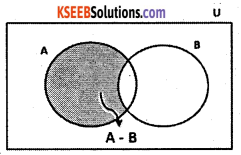Question 41.
Let A = {2,4, 6, 8} and B = (6, 8,10,12}. Find A∪B
A∪B = {2,4,6,8,10,12}.

Question 42.
Let A = {a, e, i, o, u} and B = {a, i, u}. Show that A∪B = A.
A∪B = {a,c,i,o,u} = A
∴ A∪B = AQuestion 43.
Let X = {Ram, Geeta, Akbar} be the set of students of class XI, who are in school hockey team.
Let Y = {Geeta, Divid, Ashok} be the set of students from Class XI who are in school foot ball team. Find X∪Y and interpret the set.
X∪Y= {Ram, Geeta, Akbar, Divid, Ashok}
This is the set of students from Class XI who are in the hockey team or the foot ball team or both.

Question 44.
Find the union and the intersection of each of the following pairs of sets:
(i) X ={1,3,5}, Y ={1,2,3}
(ii) A = {a,e,i,o,u},B={a,b,c}
(iii) A = {x : x is a natural number and multiple of 3}
B = {x : x is a natural number less than 6}.
(iv) A = {x:x is a natural number and 1<x≤6}
B ={x:x is a natural number and 6 < x< 10}
(v) A = {1,2,3},B = φ
(i) X∪Y = {1,2,3,5}
X∩Y = {1,3}(ii) A∪B = {a,b,c,e,i,o,u}
A∩B = {a}(iii) A = {3,6,9,12,…….. }
B = { 1,2,3,4,5,6}
∴ A∪B = {l,2,3,4,5,6,9,12, ………….. }(iv) A = {2,3,4,5,6}
B = {7,8,9}
A∪B = {2,3,4,5,6,7,8,9}
A∩B = {2,3,4,5,6,7,8,9}(v) A∪B = {1, 2,3} = A
A∩B = φ = BQuestion 45.
Let A = {a,b), B ={a,b,c}- Is A⊂B? What is A∪B?
Given sets are A = {a,b} and B = {a,b,c}.
Clearly, every element of A is the element of B, therefore A⊄B- A∪B = {a,b,c].

Question 46.
If A and B are two sets such that A‎⊂B, then what is A∪B?
Given A⊂B i.e., every element of A is contained in the set B and hence A∪B = B

Question 47.
If A ={1,2,3,4}, B ={3,4,5,6}, C ={5,6,7,8} and D = {7,8,9,10} ; find
(i) A∪B
(ii) A∪C
(iii) B∪C
(iv) B∪D
(v) A∪B∪C
(vi) A∪B∪D
(vii) B∪C∪D .
(i) A∪B = {1,2,3,4,5,6}
(ii) A∪C = {1,2,3,4,5,6,7,8}
(iii) B∪C = {3,4,5,6,7,8}
(iv) B∪D = {3,4,5,6,7,8,9,10}
(v) A∪B∪C = {1,2,3,4,5,6,7,8}
(vi) A∪B∪D = {1,2,3,4,5,6,7,8,9,10}
(vii) B∪C∪O = {3,4,5,6,7,8,9,10}Question 48.
If A = {3,5,7,9,11}, B ={7,9,11,13}, C = {11,13,15} and D = {15,17}; find
(i) A∩B
(ii) B∩C
(iii) A∩C∩D
(iv) A∩C
(v) B∩D
(vi) A∩(B∪C)
(vii) A∩D
(vii) A∩(B∪D)
(ix) (A∩B)∩(B∪C)
(x) (A∪D)∩(B∪C).
(i) A∩B = {7,9,11}
(ii) B∩C = {11,13}
(iii) A∩C∩D = φ
(iv) A∩C ={11}
(v) B∩D ={ } =φ
(vi ) A∩{B∪C) = {3,5,7,9,11}∩{7,9,11,13,15}= {7,9,11}
A∩D = φ
(viii) A∩(B∪D) = {3,5,7,9,11} ∩ {7,9,11,13,15,17} = {7,9,11}
(A∩B)∩(B∪C) = {7,9,11}∩{7,9,11,13,15} = {7,9,11}
(A∪D)∩(B∪C)
= {3,5,7,9,11,15,17} ∩ {7,9,11,13,15}.
= {7,9,11,15}

Question 49.
If A = {x : x is a natural number}, B={x:x is even natural number}, C-{x : x is an odd natural number} and D = { x: x is a prime number},find
(i) A∩B
(ii) A∩C
(iii) A∩D
(iv) B∩C
(v) B∩D
(vi) C∩D
Given A = {1,2,3,4,………………}
B = {2,4,6,8, …………..}
C = {1,3,5,7,……… }
D = {2,3,5,7,11,13, …………..}.
(i) A∩B = {2,4,6,8,……….. } = B
(ii) A∩C = {1,3,5,7,……….. } = C
(iii) A∩D = {2,3,5,7,11,13,………….}
(iv) B∩C = φ
(v) B∩D = {2}
(vi) C∩D = {3,5,7,11,13,…………}

Question 50.
Show that A∪B = A∩B implies A = B
Given A∪B = A∪B
Let x∈A.
Then x∈A∪B
x∈A∩B∴ A∩B = A∪B
⇒ y∈B
∴A ⊂B
Similarly, let y∈B .
Then y∈ A∪B
y∈A∩B ∴ A∩B = A∪B
= y∈A
∴ B⊂A
Thus, A = B.

Question 51.
Using properties of sets, show that
(i) A∪(A∩B) = A
(ii) A∩(A∪B) = A
(i) A∪(A∩B) = (A∪A)∩(A∪B) ∵ distributive law
= A∩(A∪B) = A
(ii) A∩(A∪B)
= (A∩A)∪(A∩B) ∵ distributive law
= A∪(A∩B) = A.

Question 52.
Show that A∩B = A∩C need not imply B = C
Let A = {1, 2}, B = {1, 3}, C = {1,4}.
A∩B = {1} and A∩C = {1}
A∩B = A∩C
But B≠C.

Question 53.
Let A and B be sets. If A∩X =B∩X = φ and A∪X =B∪X for some set X, show that A = B.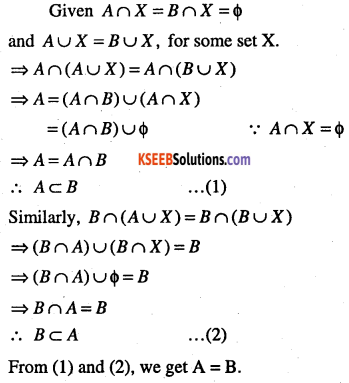Question 54.
Find sets A, B and C such that A∩B,B∩C and A∩C are non-empty sets and A∩B∩C =φ.
Let A = {a,b}, B = {b,c} and C = {a,c}.
∴ A∩B = {b}, B∩C = {c}, A∩C = {a}
⇒A∩B, B∩C and A∩C are non-empty sets Now A∩B∩C = A∩(5∩C)
= {a,b}∩{c} = φQuestion 55.
For any sets A and B, show that P(A∩B) = P(A)∩P(B)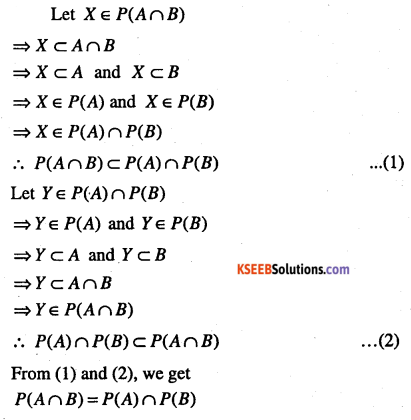Question 56.
Is it true for any sets A and B,
Let A = {φ{a}}, and B = {b}
∴ A∪B = {a,b}
P(A) = {φ,{a}}, P(B) = {φ,{b}}
and P(A∪B) = {φ,{a},{b} {a,b}}………………. (1)
and P(A)∪P(B) = {φ,{a},{b}} ………………. (2)
From (1) and (2), we get, P(A∪B) ≠P(A)∪P{B)

Question 57.
Which of the following pairs of sets are disjoint
(i) {1, 2, 3, 4} and {x : x is a natural number and 4 < x < 6}
(ii) {a,e,i,o,u} and {c,d,e,f}
(iii) {x: x is an even integer} and {x : x is an odd integer}.
(i) Given sets are {1, 2, 3,4} and {4, 5, 6}
∴ 4 is a common element.
∴ Given sets are not disjoint sets.

(ii) Given sets are {a,e,i,o,u} and {c,d,e,f}
∴ ‘e’ is a common element.
∴ Given sets are not disjoint sets.

(iii) Given sets are {0,±2,±4,- -} and {±1,+3±5,—}
∴ There is no common element.
∴ Given sets are disjoint sets.Question 58.
State whether each of the following statement is true or false. Justify your answer.
(i) {2,3,4,5} and {3,6} are disjoint sets.
(ii) {a,e,i,o,u} and {a,b,c,d} are disjoint sets.
(iii) {2,6,10,14} and {3,7,11,15} are disjoint sets
(iv) {2,6,10} and {3,7,11} are disjoint sets.
(i) Let A = {2,3,4,5} and B = {3,6}
∴ A∩B = {3} ∴ A∩B≠φ
∴Given sets are disjoint sets.(ii) Let A = {a,e,i,o,u} and B = {a,b,c,d}
A∩B = {a}.
Given sets are disjoint sets.

(iii) Given sets are A = {2,6,10,14} and B = {3,7,11,15}.
∴ A∩B =φ(iv) Given sets are disjoint sets.
Let A = {2,6,10} and B = {3, 7,11}
∴ A∩B = φ
∴ Given sets are disjoint sets.

Question 59.
Let A, B and C be the sets such that
A∪B = A∪C and A∩B = A∩C . Show, that B = C
Given A∪B=A∪C
⇒(A∪B)∩C = (A∪C)∩C
⇒(A∩C)∪(B∩C) = C
⇒(A∩B)∪(B∩C) = C ∵ A∩B = A∩C
Now, A∪B=A∪C
⇒(A∪B)∩B = (A∪C)∩B
⇒(A∩B)u(B∩B) = (A∩B)∪(C∩B)
⇒B = (A∩B)∪(B∩C)
B = C

Question 60.
If A = {3,6,9,12,15,18,21}, B = {4,8,12,16,20}, C = {2,4,6,8,10,12,14,16}, £>={5,10,15,20}. Find
(i) A-B
(ii) A-C
(iii) A-D
(iv) B – A
(v) C – A
(vi) D – A
(vii) B – C
(viii) B – D
(ix) C – B
(z) D – B
(xi) C – D
(xii) D – C.
(î) A – B={3,6,9,15,18,21)
(ii) A – C=(3,9,15,18,21)
(iii) A – D=(3,6,9,12,18,21)
(iv) B – A={4,8,16,20)
(v) C – A={2,4,8,10,14,16}
(vi) D – A=(5,10,20}
(vii) B – C={20)
(viii) B – D = (4,8,12,16)
(ix) C – B={2,6,10,14)
(x) D – B={5,10,15)
(xi) C – D={2,4,6,812,14,16}
(xii) D – C={5,15,20).

Question 61.
IV X = {a,b,c,d) and Y = (f,b,d,g), find
(i) X – Y
(ii) y – x
(iii) X∩Y.
(j) X – Y={a,c)
(ii) Y- X=(f,g)
(iii) X∩Y={b,d}

Question 62.
If R is the set of real numbers and Q is the set of rational numbers, then what is R – Q?
R – Q = set of irrational numbers.

Question 63.
Show that the following four conditions are equivalent
(i) A⊂B
(ii) A – B=φ
(iii) A ∪ B = B
(iv) A ∩ B A.
(i) ⇔ (ii):
A⊂B ⇔ All elements of A are in B
⇔ A – B = φ(ii) ⇔ (iii):
A-B = φ⇔ All elements of A are in B
⇔ A∪B = B(iii) ⇔(iv)
A∪B = B ⇔ All elements of A are in B
⇔ All elements of A are common in A and B.
⇔ A∩B = A
∴ All the four given conditions are equivalent.Question 64.
Show that for any sets A and B,
A = (A∩B)∪(A – B) and A∪(B-A) = A∪B.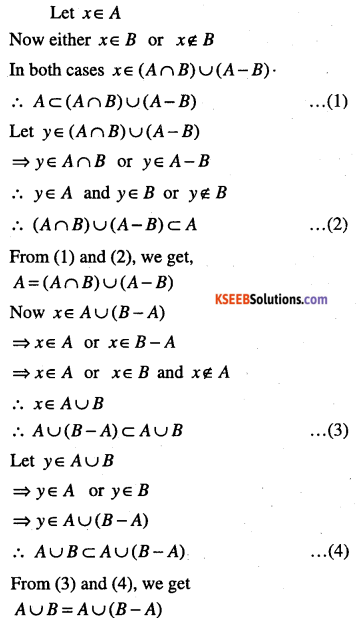Question 65.
Define complement of a set.
Let U be the universal set and A a subset of U. Then the complement of A is the set of all elements of U which are not the elements of A and is denoted by A’.
Properties:

• A∪A’
• A∩A’=φ
• (A’)’=A
• (A∪B)’= A’∩B’
• (A∩B)’ -A’∪B’

Question 66.
Let U = {1,2,3,4,5,6,7,8,9,10} and A = {1,3,5,7,9}. Find A’.
A’ = U -A = {2,4,6,8,10}Question 67.
Let U = {1, 2, 3, 4, 5, 6}, A = {2, 3} and B = {3, 4, 5}. Find A’, B’, A’∩B’,(A∪B) and hence show that (A∪B)’ = A’∩B’.
A’ = ∪ -A = { 1,4,5,6}
B’ = U-B = { 1,2,6}
A’∩B’ = {1,6}
A∩B = {2,3,4,5}
∴ (A∪B)’ = {1,6} = A’∩B’

Question 67.
If ∪={a,b,c,d,e,f ,g,h] find the complements of the following sets
(i) A={a,b,c}
(ii) B={d,e,f,g}
(iii) C ={a,c,e,g}
(iv) D = {f,g,h,a}.
(i) A’= {d,e,f,g,h}
(ii) B’ = {a,b,c,h}
(iii) C’ = {b,d,f,h]
(iv) D’ = {b,c,d,e}.

Question 68.
If U={a,b,c,d,e,f ,g,h] find the complements of the following sets
(i) A={a,b,c}
(ii) B={d,e,f,g}
(iii) C ={a,c,e,g}
(iv) D = {f,g,h,a}.
(i) A’= {d,e,f,g,h}
(ii) B’ = {a,b,c,h}
(iii) C = {b,d,f,h]
(iv) D’ = {b,c,d,e}.

Question 68.
If U = {1, 2, 3, 4, 5, 6, 7, 8, 9}, A = {2, 4, 6, 8} and B = {2,3,5,7}. Verify that
(i) (A∪B)’ = A’∩B’
(ii) (A∩B)’ = A’∪B’.
(i) A’ = {1, 3, 5, 7, 9},
(ii) B’ = {1,4, 6, 8, 9}
(iii) C’ = {b,d,f,h}
(iv) D’={b,c.d,e}

Question 69.
If U = {1, 2, 3, 4, 5, 6, 7, 8, 9}, A’ = {2, 4, 6, 8} and B’ = {2,3,5,7}. Verify that
(i) (A∪B)’ = A’∩B’
(ii) (A∩B)’ = A’∪B’.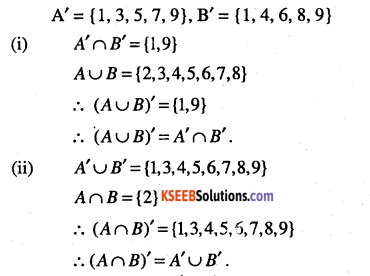Question 70.
Taking the set of natural numbers as the universal set, write down the complements of the following sets:
(i) {x: x is an even natural number}
(ii) {x : x is an odd natural number}
(iii) {x : x is positive multiple of 3}
(iv) {x : x is a prime number}
(v) {x : x is a natural number divisible by 3 and 5}
(vi) {x : x is a perfect square}
(vii) {x : x is a perfect cube}
(viii) {x : x + 5 = 8}
(ix) {x : 2x + 5 = 9}
(x) {x : x > 7}
(xi) {x : x ∈N and 2x + 1 > 10}.
(i) {x : x is an odd natural numbers}
(ii) {x : x is an even natural numbers}
(iii) {x: x is a natural number and not multiple of 3}
(iv) {x : x is a positive composite number and x = 1}
(x) {x : x∈ N and x is not divisible by 3 and 5}
(xi) {x: x∈N and x is not a perfect square}
(xii) {x:x∈N and x is not a perfect cube}
(xiii) {x:x∈2V and x≠3}
(ix) {x:x∈2V and x≠2}
(x) {1,2,3,4,5,6}
(xi) {x:x∈N and 2x + 1≤10} = {1,2,3,4}.

Question 71.
Draw appropriate Venn diagram for each of the following:
(i) (A∪B)’
(ii) A’∩B’
(iii) (A∩B’)
(iv) A’∪B’.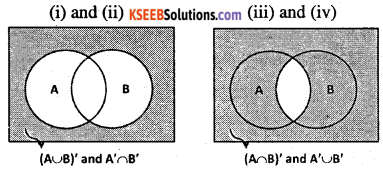Question 72.
Let U be the set of all triangles in a plane. If A is the set of all triangles with at least one angle different from 60°, what is A’?
A’ = set of equilateral triangles.

Question 73.
Fill in the blanks to make each of the following a true statement:
(i) A∪A’ = —
(ii) φ’∩A = —
(iii) A∩A’ = —
(iv) U’∩A = —
(i) A∪A’ = U
(ii) (ii) φ’∩A = A
(iii) A∩A’ = φ
(iv) U’∩A = φ.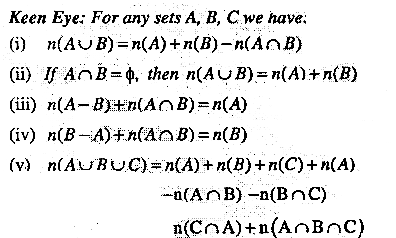Question 74.
In X and Y are two sets such that X∪Y has 50 elements, X has 28 elements and Y has 32 elements, how many elements does X∩Y have?
Given n(X∪Y) = 50; n(X) = 28; n(Y) = 32
But n(X∪Y) = n(x) + n(y)-n(X∩Y)
∴ n(X∩Y) = 28 + 32-50 = 10

Question 75.
If X and Y are two sets such that n(X) = 17, n(Y) = 23 and n(X∪Y) = 38 find n(X∩Y).
Given n(X) = 17, n(Y) = 23, n(X∪Y) = 38
But n(X∪Y) = n(X) + n(Y)-n(X∩Y)∩(X∩Y)
= 17 + 23-38 = 2

Question 76.
If X and Y are two sets such that X∪Y has 18 elements X has 8 elements and Y has 15 elements; how many elements does X∩Y have?
Given n(X∪Y) = 18, n(X) = 8, n(Y) = 15
But n(X∪Y) = n(X) + n(Y) – n(X ∩Y) n(X∩Y)
= 8 + 15-18 = 5.

Question 77.
In a group of 400 people, 250 can speak Hindi and 200 can speak English. How many people can speak both Hindi and English?
Let H = set of people speaking Hindi;
E = set of people speaking English.
Then n(H∪E) = 400; n(H) = 250; n(E) = 200
∴ n(H∩E) = n(H) = n(E)-n(H∪E)
= 250 + 200 – 400 = 50
Hence, 50 people can speak both Hindi and English.Question 78.
If S and T are two sets such that S has 21 elements, T has 32 elements and S∩T has 11 elements, how many elements does S∪T have?
Given n(5) = 21; n(T) = 32; n(S∩T) = 11
n(S∪T) = n(S) + n(T) – n(S∩T)
= 21 + 32-11 =42.

Question 79.
If X and Y are two sets such that X has 40 elements, X∪Y has 60 elements and X∩Y has 10 elements, how many elements does Y have?
Given n(X) = 40; n(X∪Y) = 60; n(X∩Y) = 10
n(Y) = n(X∪Y) + n(X∩Y)-n(X)
= 60 + 10 – 40 = 30Question 80.
In a group of 70 people, 37 like coffee, 52 like tea and each person likes atleast one of the two drinks. How many people like both coffee and tea?
Let C = set of people who like coffee
T = set of people who like tea
Then n(C∪T) = 70; n(C) = 37; n(T) = 52 n(C∩T)
= n(C) + n(J)-n(C∪T)
= 37 + 52-70 =19

Question 81.
In a group of 65 people, 40 like cricket, 10 like both cricket and tennis. How many like tennis only and not cricket? How many like tennis?
Let C = set of people who like cricket
T = set of people who like tennis.
Then n(C∪T) = 65; n(C) = 40; n(C∩T) = 10
∴ n(T) = n{C∪T) + n(C∩T)-n(C)
= 65 + 10-40 = 35
Number of people who like tennis only
n(T-C) = n(T)-n(C∩T)
= 35-10 = 25

Question 82.
In a committee, 50 people speak French, 20 speak Spanish and 10 speak both Spanish and French. How many speak atleast one of these two languages?
Let F = set of people speaking French
S = set of people speaking Spanish.
Then n(S) = 20, n(F) = 50 n(F∩S) = 10
∴ n(F∪S) = n(S) + n(F)-n(F∩S)
= 20 + 50-10 = 60
∴ 60 people speak French or Spanish or both.Question 83.
In a school there are 20 teachers who teach Mathematics or Physics. Of these, 12 teach Mathematics and 4 teach both Physics and Mathematics. How many teach Physics?
Let M = set of teachers who teach Mathematics
P = set of teachers who teach Physics
Then, n(M∪P) = 20, n(M) = 12, n(M∩P) = 4
n(P) = n(M∪P) + n(M∩P)- n(M)
= 20 + 4-12 = 12.

Question 84.
In a class of 35 students, 24 like to play cricket and 16 like to play football. Also, each student likes to play at least one of the two games. How many students like to play both cricket and football?
Let C = set of students who like cricket F = set of students who like football.
Then n(C uf) = 35, n(C) = 24, n(F)
= 16 n(C n F) = n(C) + n(F) – n(C u F)
= 24 + 16-35 = 5
∴ 5 Students like to play both games.

Question 85.
In a survey of 400 students in a school, 100 were listed as taking apple juice, 150 as taking orange juice and 75 were listed as taking both apple as well as orange juice. Find how many students were taking neither apple juice nor orange juice.
Let U = set of surveyed students
A = set of students taking apple juice
B = set of students taking orange juice.
Then n(∪) = 400; n(A) = 100; n(B) = 150;
and n(A∩B) = 75
Now n(A’∩B’) = n(A∪B)’
= n(∪) – n(A∪B)
= n(∪)-n(A)-n(B) + n(A∩S)
= 400-100-150 + 75 = 225
∴ 225 students were taking neither apple juice nor orange juice.Question 86.
In a survey of 600 students in a school. 150 students were found to be taking tea and 225 taking coffee, 100 were taking both tea and coffee. Find how many students were taking neither tea nor coffee?
Let U = set of students in a school.
A = set of students taking tea
B = set of students taking coffee
Then n(∪) = 600; n(A) = 150; n(B) = 225; n(A∩B) = 100
∴ n(Au B) = n(A) + n(B)-n(A∩B)
= 150 + 225-100 =275
Now n(A’∩B’) = n(A∪B)
= n(∪)-n(A∪5)
= 600-275 = 325
∴ 325 students were taking neither tea nor coffee.

Question 87.
In a group of students, 100 students know Hindi, 50 know English and 25 know both. Each of the students knows either Hindi or English. How many students are there in the group?
Let H = set of students, who know Hindi
E = set of students, who know English
Then n(H) = 100; n(E) = 50 and n(H∩E) = 25.
∴ n(H∪E) = n(H) + n(E)-n(H∩E)
= 100 + 50-25 = 125
Hence, 125 students are there in the group.

Question 88.
There are 200 individuals with a skin disorder, 120 had been exposed to the chemical C1, 50 to chemical C2 and 30 to both the chemicals C1 and C2. Find the number of individuals exposed to
(i) Chemical C1 but not chemical C2
(ii) Chemical C2 but not chemical C1
(iii) Chemical C1 or chemical C2.
Let U = set of individuals suffering from the skin disorder.
A = set of individuals exposed to the chemical C1.
B = set of individuals exposed to the chemical C2.
Then, n(u) = 200, n(A) = 120, n(B) = 50 and n(A∩B) = 30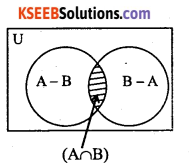(i) From the Venn diagram, we have
A = (A-B)∪(A∩B)
∴ n(A) = n(A -B) + n(A∩B)
A – B and A∩B are disjoint
∴ n(A -B) = n(A) – n(A∩B)
= 120-30 = 90
Hence, 90 individuals exposed to chemical C1 but not to chemical C2.

(ii) Similarly, B = (B – A) ∪ (A∩B)
n(B) = n(B – A) + n(A∩B)
∵ (B-A)∩(A∩B) = φ
∴ n(B-A) = n(B)-n(A∩B)
= 50-30 = 20
Hence, 20 individuals exposed to chemical C2 but not to chemical C1.

(iii) We have, n(A∪5) = n(A) + n(B) – n(A∩B)
= 120 + 50-30 = 140
Hence, 140 individuals exposed either chemical C1 or chemical C2.Question 89.
A market research group conducted a survey of 1000 consumers and reported that 720 consumers like product A and 450 consumers like product B, what is the least number that must have liked both products?
Let U = set of consumers questioned
S = set of consumers who like product A.
T = set of consumers who like the product B.
Then, n(u) = 1000, n(S) = 720, n(T) = 450
∴ n(S∪T) = n(S) + n(T)-n(S∩T)
= 720 + 450 – n(S∩T)
= 1170-n(S∩T).
Therefore, n(S∪T) is maximum when n(S∩T) is least.
But S∪T⊂U =n(S∪T)≤n(U) = 1000
So, maximum value of n(S∪T) is 1000. Thus, least value of n(S∩T) is 170.
Hence, the least number of consumers who liked both products is 170.

Question 90.
Out of 500 car owners investigated, 400 owned car A and 200 owned car B, 50 owned both A and B cars. Is this data correct?
Let U = set of car owners investigated
M = set of persons who owned car A
S = set of persons who owned car B.
Then n(u) = 500, n(M) = 400, n(S) = 200 and n(S∩M) = 50
∴ n(S∪M) = n(S) + n(M)-n(S∩M)
= 200 + 400-50 = 550
But S∪M⊂U⇒n(5∪M)≤n(U)
This is contradiction. So, the given data is incorrect.

Question 91.
A college awarded 38 medals in football, 15 in basket ball and 20 in cricket If these medals went to a total of 58 men and only three men got medals in all the three sports, how many received medals in exactly two of the three sports?
Let F = set of men who received medals in football
C = set of men who received medals in cricket.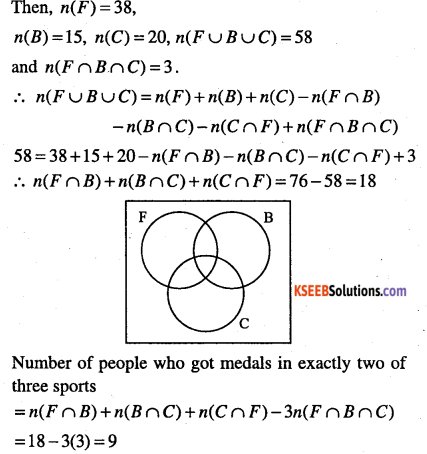Question 92.
In a survey of 60 people, it was found that 25 people read newspaper H, 26 read newspaper T, 26 read newspaper I, 9 read both H and I, 11 read both H and T, 8 read both T and I, 3 read all three news papers. Find:
(i) the number of people who read at least one of the newspapers
(ii) the number of people who read exactly one newspaper. ‘
Let H = set of people who read paper H.
I = set of people who read newspaper I
T = set of people who read newspaper T
Then, n(H) = 25, n(T) = 26, n(I) = 26, n(H∩I) = 9
n(H∩T) = 11, n(T∩I) = 8, n(H∩T∩I) = 3(i) Number of people who read atleast one newspaper
n(H∪T∪T) = n(H) + n(T) + n(I)
-n(H∩T)-n(T∩I)-n(I∩H) + n(H∩T∩I)
= 25 + 26 + 26-9-11-8 + 3 =52(ii) Number of people who read exactly one paper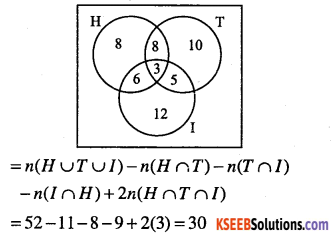Question 93.
In a survey it was found that 21 people liked product A, 26 liked product B and 29 liked product C. If 14 people liked products A and B, 12 people liked products C and A, 14 people liked products B and C and 8 liked all the three products. Find how many liked product C only.